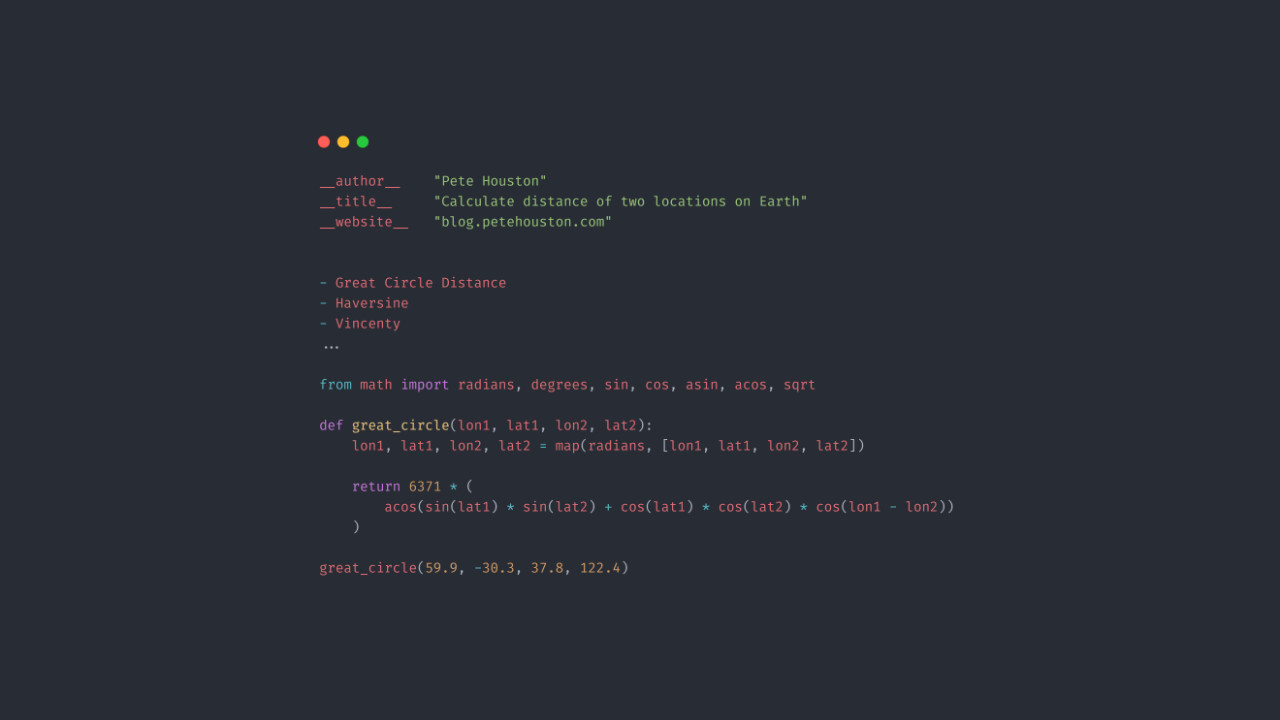# Calculate distance of two locations on Earth

1
5140We will discuss about geographic calculation in this article, and that will be how to calculate distance of two locations on Earth using Python.

Is it really possible? The answer is “YES“, not 100% accurate but pretty close, good enough to use for your apps.

Article Contents

### Mathematical Formula

In facts, you’re not the only one that think of this problem, many researchers have been working on solving this mathematical problem. As a result, there found several formula for this depending on calculation models.

• The great circle distance.
• Haversine formula.
• Vincenty formula.

Those formula will take inputs as GPS coordinates (latitude, longitude), and return the approximately distance.

If you need coordinate values, checkout this post about geocoding.

#### The Great-Circle Distance

The Great Circle distance formula computes the shortest distance path of two points on the surface of the sphere. That means, when applies this to calculate distance of two locations on Earth, the formula assumes that the Earth is spherical.

The radius `r` value for this spherical Earth formula is approximately ~6371 km.

The implementation in Python can be written like this:

``````from math import radians, degrees, sin, cos, asin, acos, sqrt

def great_circle(lon1, lat1, lon2, lat2):
lon1, lat1, lon2, lat2 = map(radians, [lon1, lat1, lon2, lat2])

return 6371 * (
acos(sin(lat1) * sin(lat2) + cos(lat1) * cos(lat2) * cos(lon1 - lon2))
)``````

If you want to use `miles`, replace `6371` with `3958.756`

#### Haversine formula

Haversine formula is also another formula to calculate distance of two locations on a sphere using the law of haversine.

Translate the formula into code, this is my Python script:

``````from math import radians, sin, cos, asin, sqrt

def haversine(lon1, lat1, lon2, lat2):
lon1, lat1, lon2, lat2 = map(radians, [lon1, lat1, lon2, lat2])

dlon = lon2 - lon1
dlat = lat2 - lat1

a = sin(dlat / 2) ** 2 + cos(lat1) * cos(lat2) * sin(dlon / 2) ** 2
return 2 * 6371 * asin(sqrt(a))``````

#### Vincenty formula

Vincenty’s formulae are two related iterative methods used in geodesy to calculate the distance between two points on the surface of a spheroid, developed by Thaddeus Vincenty (1975a). They are based on the assumption that the figure of the Earth is an oblate spheroid, and hence are more accurate than methods that assume a spherical Earth, such as great-circle distance.Wikipedia

It requires a lot of computation in Vincenty formula in order to calculate the distance of two locations on Earth; especially, the iteration to compute the delta-sigma until there is no significant change. Usually, 10e-12 (approximately ~ 0.006mm) is good enough for accuracy.

Check out the Vincenty implementation by Nathan.

### Python libraries

Most of Python geographic libraries provide those distance formula.

You can try to calculate distance of two locations (based on GPS coordinates: longitude and latitude) with following libraries:

• geopy : supports Great Circle and Vincenty formulas; additionally, it uses Karney geodesic formula as default function to calculate distance.
• geo-py : supports many methods for distance calculations in different models like sphere or ellipsoid.

### Conclusion

Depending on requirements, you will need to decide which formula is appropriate to your apps.

Generally, the Haversine formula seems to be the appropriate one most of the time.